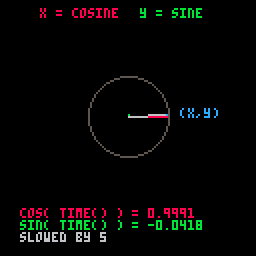# COS

``cos( x )``
 cos "cosine" x a number

The cos( x ) function is used to return the cosine of the number provided.

An example from the manual shows us how to draw a rotating dial using cos() and sin():

``````function _draw()
cls()
circ(64, 64, 20, 7)
x = 64 + cos(t()) * 20
y = 64 + sin(t()) * 20
line(64, 64, X, Y)
end``````We've slowed it down and added some more details to show what is happening here:

``````function _draw()
cls()
circ(64, 64, 20, 5)
x = 64 + cos(t()/5) * 20
y = 64 + sin(t()/5) * 20
line(64, 64, x, 64,8)  -- x-axis cosine
line(64, 64, 64, y,11) -- y-axis sine
line(64, 64, x, y,6)   -- main dial line
pset(x, y,12)          -- (x,y) point
end``````263

4 Sep 2023

`Font`